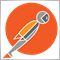# Need help on my codes for email alerts233

P.S. I have greyed out some of my attempts at it in the codes, none of my attempts has worked so far. Please help!

```#property version   "1.00"
#property strict

input bool   OpenSELL=True;
input bool   CloseBySignal=True;
input double StopLoss=20;
input double TakeProfit=1000;
input double TrailingStop=20;
input int    RSIperiod=14;
input double SellLevel=80;
input bool   AutoLot=True;
input double Risk=1;
input double ManualLots=0.1;
input int    MagicNumber=123;
input string Koment="RSIea";
input int    Slippage=10;
//---
int ticket;
int LotDigits;
double Trail,iTrailingStop;
int k;
//+------------------------------------------------------------------+
//| Expert initialization function                                   |
//+------------------------------------------------------------------+
int init()
{
k=OrdersTotal();
return(0);
}
//+------------------------------------------------------------------+
//|                                                                  |
//+------------------------------------------------------------------+
int deinit()
{
return(0);
}
//+------------------------------------------------------------------+
//|                                                                  |
//+------------------------------------------------------------------+
int start()
{
string mailtext="";
if(k != OrdersTotal()){
for(int i=0;i<OrdersTotal();i++){
string ordertyp;

double x=OrderOpenPrice(),y=OrderClosePrice(),pips;
pips =y - x;
pips =pips * 10000;
if(pips < 0)pips = - pips;
if(OrderType()==1)ordertyp="S";     // sell
mailtext=mailtext + OrderSymbol() + " "+ ordertyp + " " + DoubleToStr(OrderLots(),2) + " " + DoubleToStr(OrderOpenPrice(),5)+"\n"+TimeToStr(OrderOpenTime());
SendMail("CLOSE pr: "+DoubleToStr(OrderProfit(),2)+", bal: "+DoubleToStr(AccountBalance(),2)+", eq: "+DoubleToStr(AccountEquity(),2)+"",
"Symbol: "+Symbol()+" "+ordertyp+"   "+OrderMagicNumber()+" \n"+
"Comment: "+OrderComment()+" \n"+
"Ticket#: "+OrderTicket()+" \n"+
"Size: "+DoubleToStr(OrderLots(),2)+" \n"+
"OpenTime: "+TimeToStr(OrderOpenTime())+" \n"+
"Close Time: "+TimeToStr(OrderCloseTime())+" \n"+
"Open Price: "+DoubleToStr(OrderOpenPrice(),5)+" \n"+
"Close Price: "+DoubleToStr(OrderClosePrice(),5)+" \n"+
"Profit: "+DoubleToStr(OrderProfit(),2)+" \n"+
"Pips: "+DoubleToStr(pips,1)+" \n\n"+

"Balance: "+DoubleToStr(AccountBalance(),2)+" \n"+
"Used Margin: "+DoubleToStr(AccountMargin(),2)+" \n"+
"Free Margin: "+DoubleToStr(AccountFreeMargin(),2)+" \n"+
"Equity: "+DoubleToStr(AccountEquity(),2)+" \n"+
"Open Orders: "+DoubleToStr(OrdersTotal(),0)+" \n\n"+

"Broker: "+AccountCompany()+" \n"+
"Leverage: "+AccountLeverage()+"" );

}
/*
SendMail("Trades " + DoubleToStr(OrdersTotal(),0) + ": bal: "+DoubleToStr(AccountBalance(),2)+", eq: "+DoubleToStr(AccountEquity(),2)+"", mailtext);
k=OrdersTotal();
}

double stoplevel=MarketInfo(Symbol(),MODE_STOPLEVEL);
OrderSell=0;
for(int cnt=0; cnt<OrdersTotal(); cnt++)
{
if(OrderSymbol()==Symbol() && OrderMagicNumber()==MagicNumber && OrderComment()==Koment)
{
if(OrderType()==OP_SELL) OrderSell++;
if(TrailingStop>0)
{
iTrailingStop=TrailingStop;
if(TrailingStop<stoplevel) iTrailingStop=stoplevel;
Trail=iTrailingStop*Point;
{
}
{
ticket=OrderModify(OrderTicket(),OrderOpenPrice(),tssell,OrderTakeProfit(),0,Blue);
}
}
}
}
double rsim1=iRSI(Symbol(),1,RSIperiod,PRICE_CLOSE,0);
double rsim5=iRSI(Symbol(),5,RSIperiod,PRICE_CLOSE,1);
double rsim15=iRSI(Symbol(),15,RSIperiod,PRICE_CLOSE,1);
double rsim30=iRSI(Symbol(),30,RSIperiod,PRICE_CLOSE,1);
double rsim60=iRSI(Symbol(),60,RSIperiod,PRICE_CLOSE,1);
double rsih4=iRSI(Symbol(),240,RSIperiod,PRICE_CLOSE,1);
double rsid1=iRSI(Symbol(),1440,RSIperiod,PRICE_CLOSE,0);
double rsid2=iRSI(Symbol(),1440,RSIperiod,PRICE_CLOSE,1);
//--- open position
if(OpenSELL && OrderSell<1 && rsim1>60)
OPSELL();
/*
SendMail("New Sell Orders "+Symbol()+" Size: "+DoubleToStr(OrderLots(),2)+" OP: "+DoubleToStr(OrderOpenPrice(),3),
"Symbol: "+Symbol()+" Sell Order "+OrderMagicNumber()+" \n"+
"Comment: "+OrderComment()+" \n"+
"Ticket#: "+OrderTicket()+" \n"+
"Size: "+DoubleToStr(OrderLots(),2)+" \n"+
"OpenTime: "+TimeToStr(OrderOpenTime())+" \n"+
"Open Price: "+DoubleToStr(OrderOpenPrice(),5)+" \n"+

"Balance: "+DoubleToStr(AccountBalance(),2)+" \n"+
"Used Margin: "+DoubleToStr(AccountMargin(),2)+" \n"+
"Free Margin: "+DoubleToStr(AccountFreeMargin(),2)+" \n"+
"Equity: "+DoubleToStr(AccountEquity(),2)+" \n"+

"Broker: "+AccountCompany()+" \n"+
"Leverage: "+AccountLeverage()+"" );  */
/* SendMail("New Buy Orders "+Symbol()+" Size: "+DoubleToStr(OrderLots(),2)+" OP: "+DoubleToStr(OrderOpenPrice(),3),
"Symbol: "+Symbol()+" Buy Order "+OrderMagicNumber()+" \n"+
"Comment: "+OrderComment()+" \n"+
"Ticket#: "+OrderTicket()+" \n"+
"Size: "+DoubleToStr(OrderLots(),2)+" \n"+
"OpenTime: "+TimeToStr(OrderOpenTime())+" \n"+
"Open Price: "+DoubleToStr(OrderOpenPrice(),5)+" \n"+

"Balance: "+DoubleToStr(AccountBalance(),2)+" \n"+
"Used Margin: "+DoubleToStr(AccountMargin(),2)+" \n"+
"Free Margin: "+DoubleToStr(AccountFreeMargin(),2)+" \n"+
"Equity: "+DoubleToStr(AccountEquity(),2)+" \n"+

"Broker: "+AccountCompany()+" \n"+
"Leverage: "+AccountLeverage()+"" ); */
//--- close position by signal
if(CloseBySignal)
{
if(OrderSell>0 && rsim1>80)  CloseSell();
}
//---
return(0);
}
//+------------------------------------------------------------------+
//|                                                                  |
//+------------------------------------------------------------------+
{
double StopLossLevel;
double TakeProfitLevel;
if(StopLoss>0) StopLossLevel=Bid-StopLoss*Point; else StopLossLevel=0.0;

"Symbol: "+Symbol()+" Sell Order "+OrderMagicNumber()+" \n"+
"Size: "+DoubleToStr(LOT(),2)+" \n"+
/*"OpenTime: "+TimeToStr(OrderOpenTime())+" \n"+ */

"Broker: "+AccountCompany()+" \n"+
"Leverage: "+AccountLeverage()+"\n" );
}
//+------------------------------------------------------------------+
//|                                                                  |
//+------------------------------------------------------------------+
void OPSELL()
{
double StopLossLevel;
double TakeProfitLevel;
if(TakeProfit>0) TakeProfitLevel=Bid-TakeProfit*Point; else TakeProfitLevel=0.0;
//---
ticket=OrderSend(Symbol(),OP_SELL,LOT(),Bid,Slippage,StopLossLevel,TakeProfitLevel,Koment,MagicNumber,0,DeepPink);
SendMail("New Sell Orders "+Symbol()+" Size: "+DoubleToStr(LOT(),2)+" OP: "+DoubleToStr(Ask,3),
"Symbol: "+Symbol()+" Sell Order "+OrderMagicNumber()+" \n"+
"Size: "+DoubleToStr(LOT(),2)+" \n"+
/*"OpenTime: "+TimeToStr(OrderOpenTime())+" \n"+ */

"Broker: "+AccountCompany()+" \n"+
"Leverage: "+AccountLeverage()+"" );
}
//+------------------------------------------------------------------+
//|                                                                  |
//+------------------------------------------------------------------+
void CloseSell()
{
int  total=OrdersTotal();
for(int y=OrdersTotal()-1; y>=0; y--)
{
if(OrderSymbol()==Symbol() && OrderType()==OP_SELL && OrderMagicNumber()==MagicNumber)
{
ticket=OrderClose(OrderTicket(),OrderLots(),OrderClosePrice(),5,Black);

}
}
}
//+------------------------------------------------------------------+
//|                                                                  |
//+------------------------------------------------------------------+
{
int  total=OrdersTotal();
for(int y=OrdersTotal()-1; y>=0; y--)
{
{
ticket=OrderClose(OrderTicket(),OrderLots(),OrderClosePrice(),5,Black);
}
}
}
//+------------------------------------------------------------------+
//|                                                                  |
//+------------------------------------------------------------------+
double LOT()
{
double lotsi;
double ilot_max =MarketInfo(Symbol(),MODE_MAXLOT);
double ilot_min =MarketInfo(Symbol(),MODE_MINLOT);
double tick=MarketInfo(Symbol(),MODE_TICKVALUE);
//---
double  myAccount=AccountBalance();
//---
if(ilot_min==0.01) LotDigits=2;
if(ilot_min==0.1) LotDigits=1;
if(ilot_min==1) LotDigits=0;
//---
if(AutoLot)
{
lotsi=NormalizeDouble((myAccount*Risk)/10000,LotDigits);
} else { lotsi=ManualLots;
}
//---
if(lotsi>=ilot_max) { lotsi=ilot_max; }
//---
return(lotsi);
}
//+------------------------------------------------------------------+
```2392

BernardLin:

P.S. I have greyed out some of my attempts at it in the codes, none of my attempts has worked so far. Please help!

I looks like you have the args mixed up. The first arg is the subject and should be a short string e.g. "MyEA new order", and then you put the rest of the info into the body of the email (2nd param).

`SendMail(subject, message_body);`233

nicholi shen:

I looks like you have the args mixed up. The first arg is the subject and should be a short string e.g. "MyEA new order", and then you put the rest of the info into the body of the email (2nd param).

Hi, Shen, nice to hear from you, you mean I should just edit the SendMail function in my OPSELL() and OPBUY()? All I need to do it just to make the subject shorter? I have been scratching my head over it for a week now. It always comes with a "Mail: not enough space for 'Email subject here' " error in my journal, I would really appreciate if you can give me more detailed advice here.233

nicholi shen:

I looks like you have the args mixed up. The first arg is the subject and should be a short string e.g. "MyEA new order", and then you put the rest of the info into the body of the email (2nd param).

Hi Shen, I made the Subject in my SendMail very short per your advice, only "My new order", but when I run it, I keep getting the error message "Mail: not enough space for 'My new order' ", do you know what may cause this? Thanks!233

nicholi shen:

I looks like you have the args mixed up. The first arg is the subject and should be a short string e.g. "MyEA new order", and then you put the rest of the info into the body of the email (2nd param).

Hi Shen, I tested it again, and found that the email alert in OPBUY() and OPSELL() works fine. What seems to be causing the problem is the email alert in your old codes, during my trial, the EA only opened two buy orders, but I keep receiving tons of emails with subjects ""CLOSE pr: "+DoubleToStr(OrderProfit(),2)+", bal: "+DoubleToStr(AccountBalance(),2)+", eq: "+DoubleToStr(AccountEquity(),2)+"" from your old codes. Could you instruct me on how I should set it up so that it only sends me one email alert once a position is closed instead of keeping updating my trades even when there aren't any orders closed? Thanks very much!